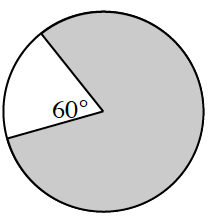### Home > INT2 > Chapter 8 > Lesson 8.4.1 > Problem8-82

8-82.

Multiple Choice: The vertex of the angle in the diagram at right is the center of the circle. What fraction of the circle is shaded?

1. $\frac { 60 } { 360 }$

1. $\frac { 300 } { 360 }$

1. $\frac { 60 } { 180 }$

1. $\frac { 120 } { 180 }$

1. none of theseB, because the portion of the shaded circle is $300^\circ$ of the total $360^\circ$.